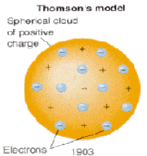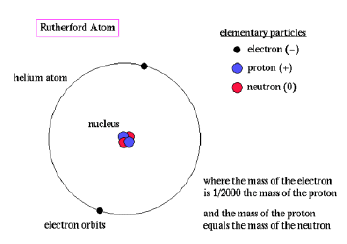#### The Development of the Model of the Atom

J. J. Thomson Model of the Atom
In the Thomson model of the atom all the mass and the entire positive charges where distributed evenly throughout the atom.
The electrons where embedded in the atom like raisins in a pudding. J.J. Thomson discovered the electron and called his model of the atom a "plum pudding" model.
In this model the atom was a positive charged core (ball) in which negatively charged particles (electrons) were embedded like raisins in a pudding.Mutual repulsion of the electrons separated them uniformly through the body of the atom.
The resulting association of positive and negative charges was reasonable and explained such properties an atomic neutrality and atomic ionization.

The Ruterord Model of the Atom
Rutherford disproved the raisin pudding model when he performed the gold foil experiment.
Rutherford’s model stated that there was an extremely dense, positively charged nucleus, in which all the mass is concentrated, surrounded by a region of negatively charged electrons.  The electrons are found in a region with a radius 100 000 x that of the nuclear radius.The Rutherford atomic model has been alternatively called the nuclear atom, or the planetary model of the atom
Probably the most serious problem with the planetary model is that an orbiting electron has a centripetal acceleration and (according to Maxwell's theory of electromagnetism) ought to lose energy by emitting electromagnetic radiation at a frequency equal to that of the orbital motion (the reciprocal of the orbital period). This radiated energy would be at the expense of the electrostatic potential energy of the electron, which would become more negative - implying that the electron approaches closer to the nucleus and experiences an increased electrostatic force. This increased force implies an increased centripetal acceleration and a higher angular velocity of the orbiting electron; the frequency of the emitted radiation would increase and the electron would spiral into the nucleus. Calculations showed that this process should happen in a small fraction of a second; in other words, the atom should not be stable !
The realization that radio waves, infrared radiation, visible light, ultraviolet radiation, X-rays and gamma rays were all forms of ELECTROMAGNETIC WAVES with different wavelengths led to a further understanding of the atom.
• These waves can be described by their wavelength, lambda, λ, amplitude and frequency, nu, υ .
• Frequency is the number of cycles of a moving wave that pass a given point per unit time.
• Units for frequency are given in cycles/second or Hertz (Hz)
• The units for wavelength are in length, the common units being meters, nanometers and Å, angstrom.The relationship between wavelength and frequency is given by Maxwell’s equation
c = λ υ
Sample problems:
1. Yellow light has a wavelength of 600 nm. What is its frequency?
Given: λ = 600 nm;      since 1 nm = 10-9 m;       then 600 nm = 6.00 x 10-7 m
c = 3.00 x 108 m/s
Required:  find the frequency, υ, of yellow light
Analysis: since c = λ υ
then  υ  =  c/λ
Solution: υ = 3.00 x 108 m/s ÷ 6.00 x 10-7 m
= 5.00 x 1014 cycles/s
Statement: The frequency of yellow light is 5.00X 1014 s-1